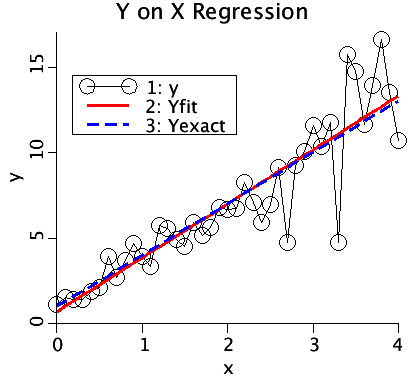# YonXregression

Model number
0362

Compute Y on X regression. Assumes y(i) = slope*x(i) +error(i) (all the error is in y.)

## Description

``` This program calculates a Y on X regression. The x values are considered exact.
Only the Y values contain error (noise). The added noise can be proportional,
i.e.,
y=Yexact*(1+Fraction*Noise), where Fraction is usually chosen 0<=Fraction<=0.25,
y=Yexact+Fraction*Noise,
where Noise is either Gaussian or Uniform with mean 0 and variance 1.```

Yexact is the dashed blue line. It graphs the exact relationship between x and Yexact.y are the circles connected by black lines. These are the Yexact values perturbed by noise (error). The noise is proportional Gaussian noise, i.e. y = Yexact*(1+Fraction*G(0,1)) where G(0,1) is Gaussian noise with mean 0 and variance 1. Fraction scales the noise and gives it a variance of Fraction*Fraction. Fraction is usually chosen to be greater than 0 and less than 0.25.

Yfit is the red line. This is a plot of the regression.

## Equations

The equations for this model may be viewed by running the JSim model applet and clicking on the Source tab at the bottom left of JSim's Run Time graphical user interface. The equations are written in JSim's Mathematical Modeling Language (MML). See the Introduction to MML and the MML Reference Manual. Additional documentation for MML can be found by using the search option at the Physiome home page.